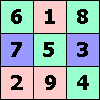ONE TO NINE by Andrew Hodges### Answers for the Extra Problems

#### This page has solutions for the problems in the original 2007 Short Books British hardback edition. The 2008 American and Canadian editions have a further Sudoku puzzle with 26 clues. Continue to a further page for solutions to the clues and the completed Sudoku.Page 322: 210 − 1 = 1023 = 11 × 93 310 − 1 = 59048 = 11 × 5368 410 − 1 = 1048575 = 11 × 95325 510 − 1 = 9765624 = 11 × 887784 610 − 1 = 60466175 = 11 × 5496925... 999999 breaks down into prime factors as 33 × 7 × 11 × 13 × 37. So out of the decimals for fractions 1/p, p a prime, only 1/3, 1/7, 1/11, 1/13 and 1/37 can recur with period 6. 9999 = 32 × 11 × 101. So only 1/3, 1/11 and 1/101 recur with period 4. This question shows the difficulty of finding the factors of even a 7-figure number like 9999999. You may find it helpful to use my factorisation applet. 9999999 = 32 × 239 × 4649. So only 1/3, 1/239 and 1/4649 recur with period 7. In fact 1/239 = .0041841 recurring, 1/4649 = .0002151 recurring Only 1/21649 and 1/513239 recur in groups of 11. To find 1/5 in binary notation, you could do long division in binary. However, it is easier to use the 'geometric progression' principle. 5 must divide into 24 − 1, by Fermat's theorem, and indeed it does since 5 × 3 = 15. Now using r = 1/16, the formula for geometric progressions gives: 1/15 = 1/16 + 1 /162 + 1/163... = .0001000100010001... So 1/5 = 3/15 = .0011001100110011... A calculator working in binary will therefore not be able to hold 1/5 exactly. For such a calculator, 1/5 is just as awkward as 1/3, which a ten-based calculator cannot store exactly. Is this to be thought of as a fault or defect of binary arithmetic? Not in any deep sense, but from a practical point of view it is a disadvantage to have inconsistency with ten-based working. My impression is that calculators given as software on computers now store numbers as if they were working in decimal notation. The Power Mac of the 1990s was unusual in working directly in binary. The deeper point illustrated by all this is Turing's principle that a computer, as a universal machine, can perform calculation in any base you like, just by running the right software. The binary basis of its hardware is not very important. To see how the calculator is actually storing numbers, try entering 1/3, then mulitplying by 10 repeatedly, subtracting off the 3 at the beginning each time. It will reveal a string of 3's of a finite length, determined by the precision used. This problem amounts to asking what is the first power of 6 that is congruent to 1, modulo 13. Fermat tells us that 612 has this property. 62 = 10 modulo 13, so it cannot be true that 66 = 1 modulo 13 as this would imply 103 = 1 modulo 13 which is not true. This also means that 63 is not 1 modulo 13, and 62, 64 can't be 1 modulo 13 either. Explicitly, 1/13 in base-6 is .024340531215 recurring = 167444795 /(612 − 1). The argument for base-7 is the same. By an extension of this argument, you can see that in base 9, 1/13 has a recurring period of 3 (actually .062 recurring), in base 5 of length 4 (actually .0143 recurring), and in base 8 of length 4 (actually .0473 recurring). This is an extension of the idea of a geometric series. Again write r for 1/10. Then we want to show that 1/89 = F0 × r + F1 × r2 + F2 × r3 + F3 × r4... where F0, F1, F2, F3... are the Fibonacci numbers 0, 1, 1, 2... Multiply both sides by (1 − r − r2). All the terms cancel, because of the property of the Fibonacci numbers, except for F0 × r + F1 × r2 − F0 × r2 and this is just r2 = 1/100. But (1 − r − r2) is just 89/100, so this verifies the equation. Putting r = 1/10000 instead, we find that 1/9899 = .000102030508132134... For a more direct approach to Fermat's Little Theorem, take the example of p=7, and look at the 7th row of the Pascal triangle, which is: 1, 7, 21, 35, 35, 21, 7, 1. It is noticeable that all the entries, apart from the 1's at the ends, are divisible by 7. Is this a fluke? No: there is good reason, which depends on knowing the connection with the factorials. The 35, for instance, arises as 7!/(3! × 4!), representing the number of ways of choosing 3 things out of 7. The 7! has a factor of 7 in it, but 3! and 4! do not. So the factor of 7 must be present in the number 7!/(3! × 4!), as indeed it is. The same argument applies to any number in the pth row, when p is a prime. (It does not apply if p is not a prime). The numbers in the pth row of the Pascal triangle sum to 2p. Subtracting the two 1's at the ends, the number 2p − 2 must be divisible by p, and this is Fermat's Little Theorem.